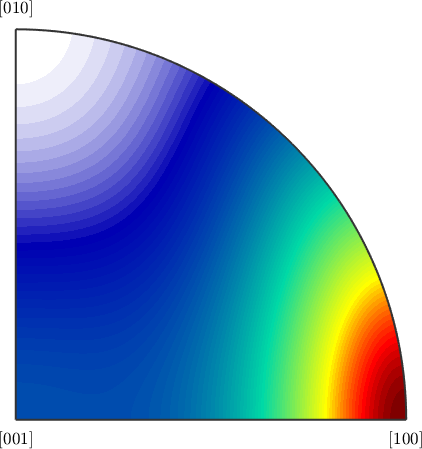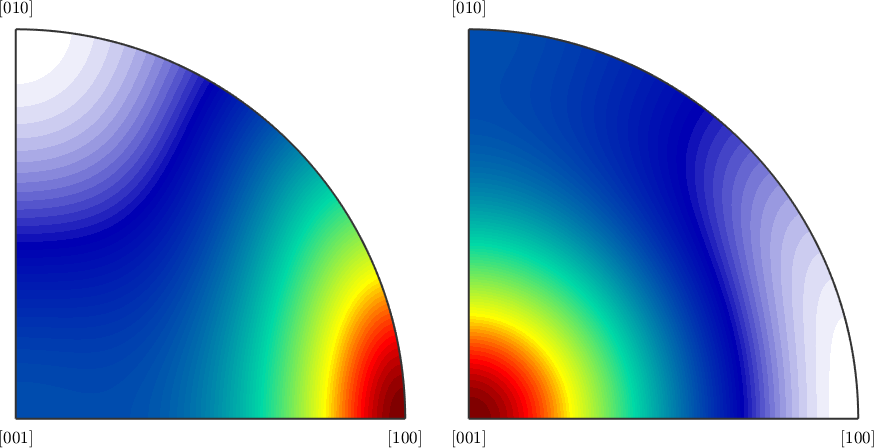Alignment of the Crystal Axes edit page

Default is $$\vec c$$ axis of highest symmetry.

TODO: Explain the default setting in more detail.

## Switching between different Alignment Options

Since, especialy for lower symmetry groups, different conventions for aligning the crystal axes are used it might be necessary to transform data, e.g, orientations or tensors, from one convention into another. This can be done using the command transformReferenceFrame as it illustrated below.

First we import the stiffness tensor Forsterite with respect to the axes alignmentLet us now consider a different setup of the Forsterite symmetry, where the $$\vec a$$ axis is the longest and the $$\vec c$$-axis is the shortest.

In order to represent the stiffness tensor C with respect to this setupt we use the command transformReferenceFrame.